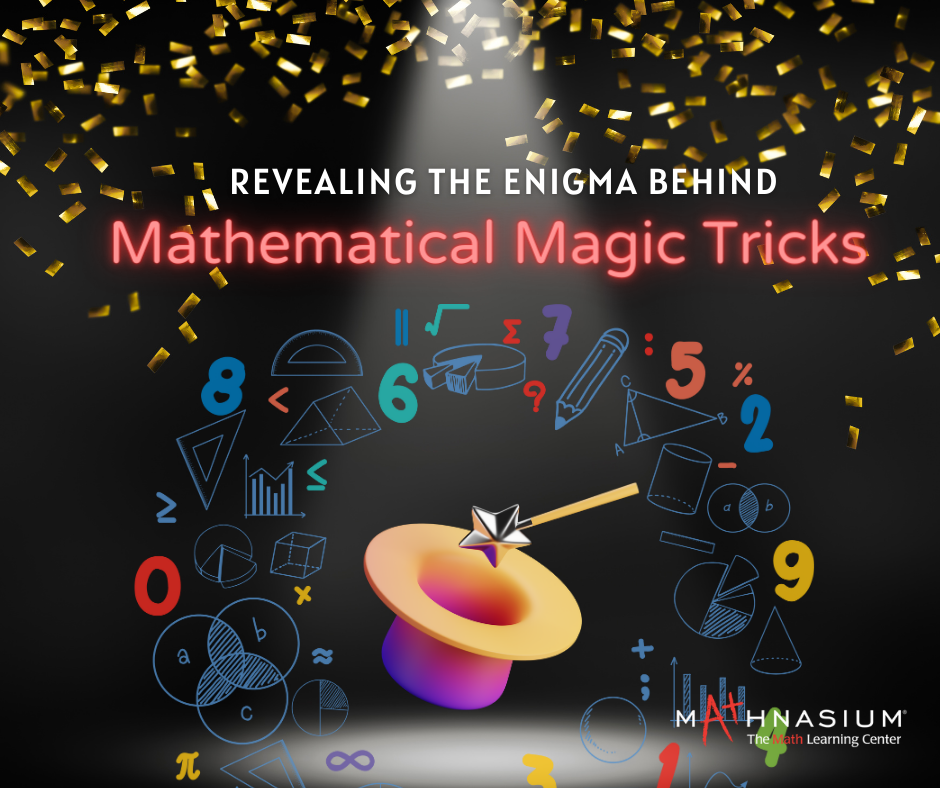877-601-6284
Get Started by Finding a Local Center

# Unveiling the Mystique of Math Magic Tricks

Aug 22, 2023 | West ChesterMagic tricks have always captivated audiences with their spellbinding illusions and mysterious allure. But did you know that mathematics, often associated with logic and equations, can also be the source of enchantment? In this blog, we'll explore the world of math magic tricks – delightful feats that merge mathematical principles with the art of illusion to amaze and entertain your friends and family.

The Uncanny Prediction:

Here's a trick that will leave your audience bewildered. Ask a friend to choose any number and perform the following steps secretly:

a. Multiply the chosen number by 2.

b. Add 8 to the result.

c. Divide the total by 2.

d. Finally, subtract the original chosen number.

No matter the starting number, the outcome will always be 4! The secret here is algebraic manipulation. The series of operations ensures that the initial number cancels out, leaving the mystifying and consistent result of 4.

The Enchanted Number 1089:

Prepare a two- or three-digit number with distinct digits (e.g., 375 or 684). Reverse the digits to create a new number (573 or 486), and then subtract the smaller from the larger number. Now, reverse the result and add it to the original difference. Astonishingly, you'll always end up with the number 1089! This mathematical magic trick demonstrates the power of numbers and their unique properties.

The Multiplying by 9 Trick:

This trick is a fun way to impress your friends with quick mental calculations. To multiply any number by 9, follow these steps:

a. Write down the number.

b. Subtract 1 from the original number.

c. Add the difference to the original number, placing the sum after the difference.

The result will always be the original number followed by digits that add up to 9. For instance, multiplying 7 by 9 means you'll get 63 (7 + 56).

The Eleven Fingers Trick:

This is more of a joke than a trick, yet it really mystifies many people. Tell the group that you have 11 fingers, counting your thumbs. Using your right forefinger as a pointer touch each finger of the left hand, counting "one, two, three, four, five." Then your left forefinger counts the right fingers: "Six, seven, eight, nine, ten." "Strange," you say, "I thought I had eleven. Let's try again." You start counting backward, pointing to the fingers of the right hand. "Ten, nine, eight, seven, six," then stop, hold up your left hand and say: "And five are eleven!" Executed rapidly, this clever method of counting is very deceptive.

Math magic tricks reveal the enchanting side of mathematics, transforming seemingly complex principles into captivating and entertaining illusions. By mastering these tricks, you can amaze your friends and family while gaining a deeper appreciation for the hidden wonders of numbers and calculations. So, whether you're folding fingers or predicting numbers, math magic tricks offer a delightful fusion of logic and illusion that will leave everyone spellbound.

Locations near

Could not find Center, try again